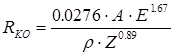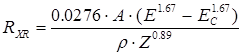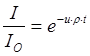# Calculate Electron and X-ray Ranges

### Calculate Electron and X-ray Ranges

This menu pulls up a modeless dialog that can do three related range calculations. First given a specified composition (using either atom or weight fraction input), it will calculate the electron range (Kanaya-Okayama) in microns for a given density and incident beam energy, second, it will calculate the x-ray production range in microns based on the above and a specified element and x-ray line, third, it will calculate the x-ray transmission, in unit fraction, for the above conditions given a specified thickness.

The three equations (Goldstein, et. al., 1981) used for these calculation are shown here, where A is average atomic weight and Z is average atomic number (calculated using mass fraction averaging) and E is beam energy and EC is critical excitation energy both in keV and t is thickness in cm and ρ is density in gm/cm3:

1.2.3.